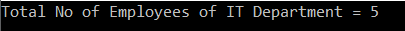# Linq Count Method in C# with Examples

In this article, I am going to discuss the Linq Count Method in C# with examples. Please read our previous article before proceeding to this article where we discussed the Linq Average Aggregate method with some examples. As part of this article, we are going to discuss the following pointers.

1. What is Linq Count Method in C#?
2. Multiple examples using both Method and Query syntax.
##### What is Linq Count Method in C#?

The Linq Count Method used to return the number of elements present in the collection or the number of elements that have satisfied a given condition.

There are two overloaded versions of the Linq Count() extension method is available as shown below.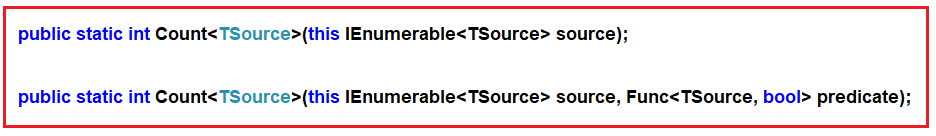As you can see from the above definitions, the first overloaded version does not take any parameter and it simply returns the count of the number of elements present in the collections. On the other hand, the second overloaded version takes one predicate as a parameter and then it returns the count of the number of elements which have satisfied the given condition which we can specify using a lambda expression or by using a predicate function.

The return type of Count() function is always going to be int. Let us understand the Count() method with some examples.

##### Example1:

The following example returns the number of elements present in the collection.

```using System;
using System.Linq;
namespace LINQDemo
{
class Program
{
static void Main(string[] args)
{
int[] intNumbers = new int[] { 60, 80, 50, 90, 10, 30, 70, 40, 20, 100 };

//Using Method Syntax
int MSCount = intNumbers.Count();

//Using Query Syntax
var QSCount = (from num in intNumbers
select num).Count();

Console.WriteLine("No of Elements = " + MSCount);
}
}
}
```

Output: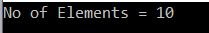Note: We don’t have any operator called Count in Linq to write the query syntax. So here we need to use the mixed syntax.

##### Example2: Count with filter

Now we need to return the number of elements present in the collection which are greater than 40.

```using System;
using System.Linq;
namespace LINQDemo
{
class Program
{
static void Main(string[] args)
{
int[] intNumbers = new int[] { 60, 80, 50, 90, 10, 30, 70, 40, 20, 100 };

//Using Method Syntax
int MSCount = intNumbers.Where(num => num > 40).Count();

//Using Query Syntax
var QSCount = (from num in intNumbers
where num > 40
select num).Count();

Console.WriteLine("No of Elements = " + MSCount);
}
}
}```

Output: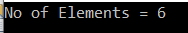##### Working with Complex Type:

We are going to work with the following Employee class.

```using System.Collections.Generic;
namespace LINQDemo
{
public class Employee
{
public int ID { get; set; }
public string Name { get; set; }
public int Salary { get; set; }
public string Department { get; set; }

public static List<Employee> GetAllEmployees()
{
List<Employee> listStudents = new List<Employee>()
{
new Employee{ID= 101,Name = "Preety", Salary = 10000, Department = "IT"},
new Employee{ID= 102,Name = "Priyanka", Salary = 15000, Department = "Sales"},
new Employee{ID= 103,Name = "James", Salary = 50000, Department = "Sales"},
new Employee{ID= 104,Name = "Hina", Salary = 20000, Department = "IT"},
new Employee{ID= 105,Name = "Anurag", Salary = 30000, Department = "IT"},
new Employee{ID= 106,Name = "Sara", Salary = 25000, Department = "IT"},
new Employee{ID= 107,Name = "Pranaya", Salary = 35000, Department = "IT"},
new Employee{ID= 108,Name = "Manoj", Salary = 11000, Department = "Sales"},
new Employee{ID= 109,Name = "Sam", Salary = 45000, Department = "Sales"},
new Employee{ID= 110,Name = "Saurav", Salary = 25000, Department = "Sales"}
};

return listStudents;
}
}
}
```

We create the Employee class with four properties such as ID, Name, Salary, and Department along with the GetAllEmployees() which in turn going to return the list of all the employees.

##### Example3:

The following example returns the number of employees.

```using System;
using System.Linq;
namespace LINQDemo
{
class Program
{
static void Main(string[] args)
{
//Using Method Syntax
var MSCount = Employee.GetAllEmployees().Count();

//Using Query Syntax
var QSCount = (from emp in Employee.GetAllEmployees()
select emp).Count();

Console.WriteLine("Total No of Employees = " + QSCount);
}
}
}
```

Output: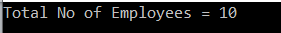##### Example4:

The following example returns the number of employees in the IT department.

```using System;
using System.Linq;
namespace LINQDemo
{
class Program
{
static void Main(string[] args)
{
//Using Method Syntax
var MSCount = Employee.GetAllEmployees()
.Where(emp => emp.Department == "IT")
.Count();

//Using Query Syntax
var QSCount = (from emp in Employee.GetAllEmployees()
where emp.Department == "IT"
select emp).Count();

Console.WriteLine("Total No of Employees of IT Department = " + QSCount);